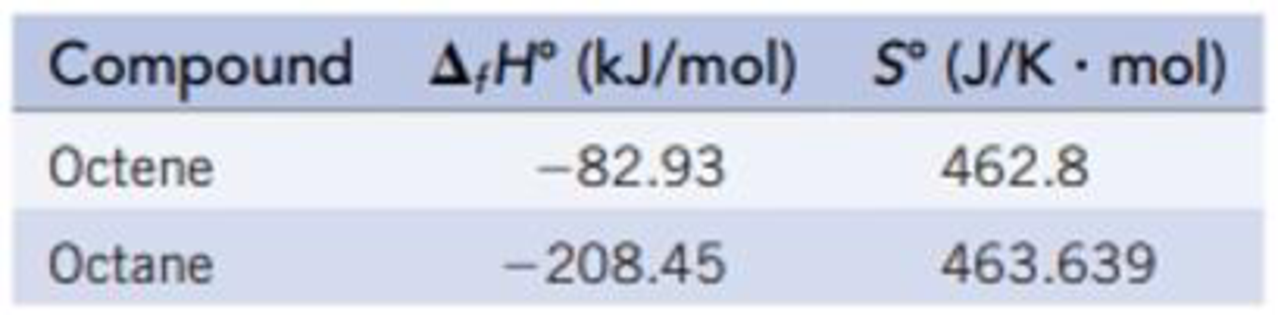Chapter 18, Problem 54GQ

Chapter
Section
Textbook Problem

Hydrogenation, the addition of hydrogen to an organic compound, is an industrially important reaction. Calculate ΔrH°, ΔrS°, and ΔrG° for the hydrogenation of octene, C8H16, to give octane, C8H19 at 25 °C. Is the reaction product- or reactant-favored at equilibrium?C8H16(g) + H2(g) → C8H18(g)Along with data in Appendix L, the following information is needed for this calculation.Interpretation Introduction

Interpretation:

The value of ΔrH°,ΔrS° and ΔrG for the hydrogenation of octene should be calculated.

Concept introduction:

The Gibbs free energy or the free energy change is a thermodynamic quantity represented by ΔrG. It is related to entropy and entropy by the following expression,

ΔrG=ΔrHTΔrS

Here, ΔrH is the change in enthalpy and ΔrS is the change in entropy.

Explanation

The value of ΔrH°, ΔrS° and ΔrG for the hydrogenation of octane is calculated below.

Given:

C8H16(g)+H2(g)C8H18(g)ΔfH°(kJ/mol)82.930208.45S(J/Kmol)462.8+130.7463.639

ΔrH°=nΔfH°(products)nΔfH°(reactants)=[(1 mol C8H18(g)/mol-rxn)ΔfH°[C8H18(g)][(1 mol C8H16(g)/mol-rxn)ΔfH°[C8H16(g)]+(1 mol H2(g)/mol-rxn)ΔfH°[H2(g)]] ]

Substituting the values,

ΔrH°=[(1 mol C8H18(g)/mol-rxn)(208.45 kJ/mol)[(1 mol C8H16(g)/mol-rxn)(82.93 kJ/mol)+(1 mol H2(g)/mol-rxn)(0 kJ/mol)] ]=291

Still sussing out bartleby?

Check out a sample textbook solution.

See a sample solution

The Solution to Your Study Problems

Bartleby provides explanations to thousands of textbook problems written by our experts, many with advanced degrees!

Get Started